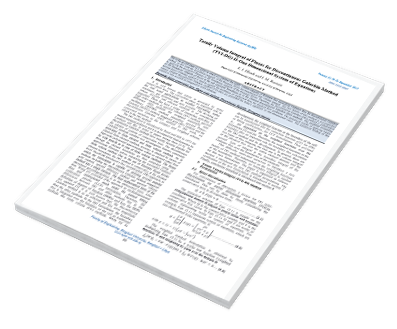# Totally Volume Integral of Fluxes for Discontinuous Galerkin Method (TVI-DG) II-One Dimensional System of Equations

## Keywords:

Scalar conservation laws; Higher order methods, Discontinuous Galerkin; Divergence theorem

## Abstract

This is the second paper in a series in which we construct the totally volume integrals of Riemann flux mimicking Godunov flux for discontinuous Galerkin method. In this work the boundaries integrals of the Riemann fluxes are transformed into volume integral. The new family of DG method is accomplished by applying the divergence theorem to the boundaries integrals of the Riemann fluxes. Therefore, the discontinuous Galerkin (DG) method is independent on the boundaries integrals of fluxes at the cell (element) boundaries as in the classical discontinuous Galerkin (DG) methods. The modified streamline upwind Petrov-Galerkin method is used to capture the oscillation of unphysical flow for shocked flow problems. The numerical results of applying totally volume integral discontinuous Galerkin method (TVI-DG) are presented for Euler's equations in one-dimensional cases. The numerical finding of this scheme is very accurate as compared to exact solutions.2017-09-03

## How to Cite

Rustum, E. I. E. (2017). Totally Volume Integral of Fluxes for Discontinuous Galerkin Method (TVI-DG) II-One Dimensional System of Equations. Libyan Journal for Engineering Research, 1(2). Retrieved from https://journals.uob.edu.ly/LyJER/article/view/472

Articles